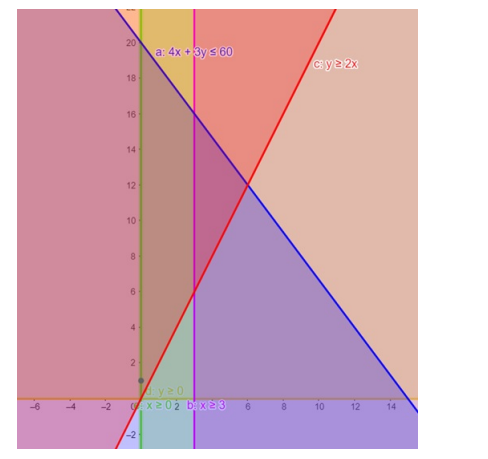# Solve the given inequalities

Question:

Solve the given inequalities $4 x+3 y \leq 60, y \geq 2 x, x \geq 3, x \geq 0, y \geq 0$ graphically in two - dimensional plane.

Solution:

The graphical representation of $4 x+3 y \leq 60, y \geq 2 x, y \geq 0$

$x \geq 3, x \geq 0$ is given by common region in the figure below.

$4 x+3 y \leq 60$ ........(1)

$y \geq 2 x \ldots \ldots(2)$

$x \geq 0$.............(3)

$y \geq 0$ .........(4)

$x \geq 3$ ...........(5)

Inequality (1) represents the region below line $4 x+3 y=60$ (including the line $4 x+3 y=60$ ).

Inequality (2) represents the region above line $y=2 x$ (including the line $y=2 x$ ).

Inequality (3) represents the region in front of line $x=0$ (including the line $x=0$ ).

Inequality (4) represents the region above line $y=0$ (including the line $y=0$ ).

Inequality (5) represents the region in front of line $x=3$ (including the line $x=3$ )

Therefore,every point in the common shaded region including the points on the respective lines represents the solution for the given inequalities.

This can be represented as follows,